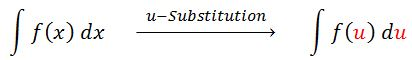# U-Substitution

### U-Substitution

You may start to notice that some integrals cannot be integrated by normal means. Therefore, we introduce a method called U-Substitution. This method involves substituting ugly functions as the letter "u", and therefore making our integrands easier to integrate. We will use this technique to integrate many different functions such as polynomial functions, irrational functions, trigonometric functions, exponential functions and logarithmic functions. We will also integrate functions with a combination of different types of functions.
Basic concepts: Chain rule, Antiderivatives,

#### Lessons

Pre-requisite:
* Differential Calculus –"Chain Rule"
* Integral Calculus –"Antiderivatives"
Note:
The main challenge in using the $u-Substitution$ is to think of an appropriate substitution.
- Question: how to choose $u$?
- Answer: choose $u$ to be some function in the integrand whose differential also occurs!
hint:
$u$ is usually the inside of a function, for example:
- the inside a power function: $( u )^{10}$
- the inside a radical function: $\sqrt{u}$
- the inside of an exponential function: $e^u$
- the inside of a logarithmic function: $\ln$? $(u)$
- the inside of a trigonometric function: $\sin$ $(u)$
• Introduction
Introduction to u-Substitution
$\cdot$ What is $u$-Substitution?$\cdot$ Exercise: Find $\int (5x^4-6x) \cos (x^5-3x^2)dx$.
- How to pick "$u$"?
- How to verify the final answer?

• 1.
Integrate: Polynomial Functions
$\int-7x(6x^2+1)^{10}dx$

• 2.
a)
$\int\frac{x^6}{{^3}\sqrt{(8-5x^7)^2}}dx$

b)
$\int\sqrt{6-3x}$ $dx$

• 3.
Integrate: Exponential Functions
$\int e^{2x}dx$

• 4.
Integrate: Logarithmic Functions
a)
$\int \frac{(\ln x)^3}{x}dx$

b)
$\int \frac{dx}{x \ln x}$

• 5.
Integrate: Trigonometric Functions
a)
$\int \sin ^3 x \cos x\; dx$

b)
$\int \sec ^2 x(\tan x-1)^{100}\;dx$

• 6.
Not-So-Obvious U-Substitution
a)
$\int \sqrt{x^3-8}x^5dx$

b)
$\int {^3}\sqrt{1+x^2}x^5dx$

c)
$\int \frac{1+x}{1+x^2}dx$

d)
$\int \cot x$ $dx$

• 7.
Evaluate Definite Integrals in Two Methods
Evaluate: $\int_{-1}^{2} \sqrt{6-3x} dx$
a)
Introduction to definite integrals.

b)
Method 1: evaluate the definite integral in terms of "$x$".

c)
Method 2: evaluate the definite integral in terms of "$u$".

d)
Method 1 VS. Method 2.

• 8.
Evaluate Definite Integrals
Evaluate: $\int_{0}^{\frac{\pi}{3}} \frac{\sin \theta}{\cos ^2 \theta}d \theta$

• 9.
Definite Integral: Does Not Exist (DNE)
Evaluate: $\int_{1}^{5} \frac{dx}{(x-3)^2}$